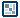## Question of the WeekExperiments with Perspective
by ilyas usal

Introduction
OK people. Now that you've all read and understood and experimented with the perspective tutorial (if not, click here), and that you feel that you have a good grasp on the concept, we can start the fun stuff. I am going to try and show you how you can make this simple idea evolve into something really cool.

One more thing: this is not a tutorial, so I won't explain my code so much. It is not very complicated, and I'm sure that if you experiment with the key variables you'll see what they do and how to use them.

First experiment: a starfield

[ a starfield, generated randomly ]

Steps to Create Animation
Now one dot is fine, several dots is better. Add a little bit of randomness and it's great. Let's look at what we have to change compared to the last code we had.

1. First we want the dots to stay still, so we can remove the `EnterFrame` part. Then we want to have several stars, so we have to duplicate our clip. Finally, we want their depth to be random, so we are going to assign a random `z` value to our duplicated clips.

```onClipEvent (load) {    maxDepth=1000;    z=Math.round(Math.random()*maxDepth);    fl=300;    xcenter=175;    ycenter=175;    offx=(startx-xcenter);    offy=(starty-ycenter);    scale=fl/(fl+z);    _x=xcenter+offx*scale;    _y=ycenter+offy*scale;    _xscale=_yscale=100*scale; }```

When the clip will be duplicated, it will resize and position depending on variables that are either fed to it (`startx`) or generated by the clip itself (`z, scale`). Then we have to duplicate this clip a certain number of times. We are going to use the _root to do this, which has the advantage to work with both Flash 5 and Flash MX (I think...).

Enter this code on the first frame of the _root:

```nbClip=50; for (i=0;i<nbClip;i++){    duplicateMovieClip(ball,"ball"+i,i);    mc=_root["ball"+i];    mc.startx=Math.round(Math.random()*300)-50;    mc.starty=Math.round(Math.random()*300)-50; } ```

The key variables here are:

1. maxDepth: the maximal depth
2. z: depth, randomly set when the clip loads
3. fl: focal length
4. xcenter, ycenter: coordinates of the horizon point
5. startx, starty: coordinates of the star in space, set during the duplication
6. offx, offy: offset of the star compared to the horizon point

Change them to see what they do.

Now there are a few things we can improve as you can see. First, bigger stars should be on top of smaller ones. Logical. And also small stars should be less luminous than big ones. So we are going to add a few lines at the end of the code we put on the `ball` movie clip.

[ a bit of _alpha here and there ]

1. The new code is:

```onClipEvent (load) {    maxDepth=1000;    z=Math.round(Math.random()*maxDepth);    fl=300;    xcenter=175;    ycenter=175;    offx=(startx-xcenter);    offy=(starty-ycenter);    scale=fl/(fl+z);    _x=xcenter+offx*scale;    _y=ycenter+offy*scale;    _xscale=_yscale=100*scale;    _alpha=scale*100;    this.swapDepths(scale*1000); }```

You can remove the `_alpha` line, to see what the `swapDepths` does.

Last thing, it would be nice to be able to change the view a bit. To do so, we can either move the horizon point, or move the stars. I'll show you how to move the horizon point, the other one I let you search a bit :)

[ a starfield, with perspective ]

1. So the duplication process remains unchanged. What we need to change is the `EnterFrame` handler of the stars, because they have to check all the time where is the horizon point. We will make the horizon point follow the mouse, by the way.

2. The complete code of the star will be:

```onClipEvent (load) {    maxDepth=1000;    z=Math.round(Math.random()*maxDepth);    fl=300;    scale=fl/(fl+z);    _xscale=_yscale=100*scale;    _alpha=scale*scale*100;    this.swapDepths(scale*1000); } onClipEvent (enterFrame){    var xcenter=_root._xmouse;    var ycenter=_root._ymouse;    var offx=(startx-xcenter);    var offy=(starty-ycenter);    _x=xcenter+offx*scale;    _y=ycenter+offy*scale; }```

There you go! A nice starfield with perspective. I hope you enjoyed this experiment, and don't forget to check this section regularly because there will be some more coming.

Also, if you've done something cool with this experiment, please show us, either on the board, or send them directly to me, I'll be glad to have a look at it.